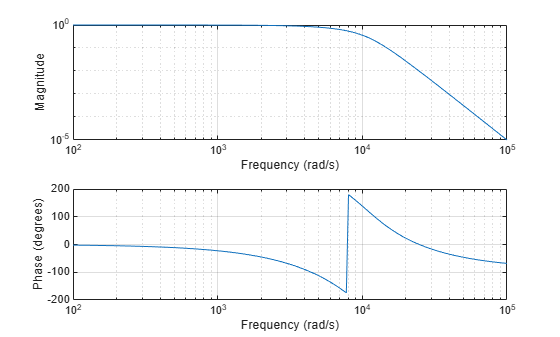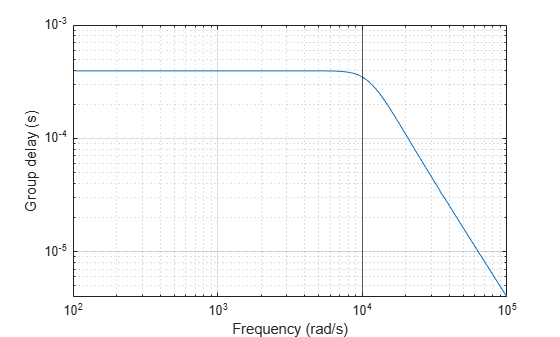besself

Bessel analog filter design

Description

example

[b,a] = besself(n,Wo) returns the transfer function coefficients of an nth-order lowpass analog Bessel filter, where Wo is the angular frequency up to which the filter's group delay is approximately constant. Larger values of n produce a group delay that better approximates a constant up to Wo. The besself function does not support the design of digital Bessel filters.

example

[b,a] = besself(n,Wo,ftype) designs a lowpass, highpass, bandpass, or bandstop analog Bessel filter, depending on the value of ftype and the number of elements of Wo. The resulting bandpass and bandstop designs are of order 2n.

[z,p,k] = besself(___) designs a lowpass, highpass, bandpass, or bandstop analog Bessel filter and returns its zeros, poles, and gain. This syntax can include any of the input arguments in previous syntaxes.

[A,B,C,D] = besself(___) designs a lowpass, highpass, bandpass, or bandstop analog Bessel filter and returns the matrices that specify its state-space representation.

Examples

collapse all

Design a fifth-order analog lowpass Bessel filter with approximately constant group delay up to $1{0}^{4}$ rad/second. Plot the magnitude and phase responses of the filter using freqs.

[b,a] = besself(5,10000);
freqs(b,a)Compute the group delay response of the filter as the derivative of the unwrapped phase response. Plot the group delay to verify that it is approximately constant up to the cutoff frequency.

[h,w] = freqs(b,a,1000);
grpdel = diff(unwrap(angle(h)))./diff(w);

clf
semilogx(w(2:end),grpdel)
ylabel('Group delay (s)')Design a 12th-order bandpass Bessel filter with the passband ranging from 300 rad/s to 500 rad/s. Compute the frequency response of the filter.

[b,a] = besself(6,[300 500],'bandpass');

[h,w] = freqs(b,a);

Plot the magnitude and phase responses of the filter. Unwrap the phase response to avoid ${180}^{\circ }$ and ${360}^{\circ }$ jumps and convert it from radians to degrees. As expected, the phase response is close to linear over the passband.

subplot(2,1,1)
plot(w,20*log10(abs(h)))
ylabel('Magnitude')
subplot(2,1,2)
plot(w,180*unwrap(angle(h))/pi)
ylabel('Phase (degrees)')Input Arguments

collapse all

Filter order, specified as an integer scalar. For bandpass and bandstop designs, n represents one-half the filter order.

Data Types: double

Cutoff frequency, specified as a scalar or a two-element vector. A cutoff frequency is an upper or lower bound of the frequency range in which the filter's group delay is approximately constant. Cutoff frequencies must be expressed in radians per second and can take on any positive value.

• If Wo is scalar, then besself designs a lowpass or highpass filter with cutoff frequency Wo.

• If Wo is a two-element vector [w1 w2], where w1 < w2, then besself designs a bandpass or bandstop filter with lower cutoff frequency w1 and higher cutoff frequency w2.

Data Types: double

Filter type, specified as:

• 'low' — a lowpass filter with cutoff frequency Wo. 'low' is the default for scalar Wo.

• 'high' — a highpass filter with cutoff frequency Wo.

• 'bandpass' — a bandpass filter of order 2n if Wo is a two-element vector. 'bandpass' is the default when Wo has two elements.

• 'stop' — a bandstop filter of order 2n if Wo is a two-element vector.

Output Arguments

collapse all

Transfer function coefficients of the filter, returned as row vectors of length n + 1 for lowpass and highpass filters and 2n + 1 for bandpass and bandstop filters. The transfer function is expressed in terms of b and a as

$H\left(s\right)=\frac{B\left(s\right)}{A\left(s\right)}=\frac{\text{b(1)}\text{\hspace{0.17em}}{s}^{n}+\text{b(2)}\text{\hspace{0.17em}}{s}^{n-1}+\cdots +\text{b(n+1)}}{\text{a(1)}\text{\hspace{0.17em}}{s}^{n}+\text{a(2)}\text{\hspace{0.17em}}{s}^{n-1}+\cdots +\text{a(n+1)}}.$

Data Types: double

Zeros, poles, and gain of the filter, returned as two column vectors of length n (2n for bandpass and bandstop designs) and a scalar. The transfer function is expressed in terms of z, p, and k as

$H\left(s\right)=\text{k}\frac{\left(s-\text{z(1)}\right)\text{\hspace{0.17em}}\left(s-\text{z(2)}\right)\cdots \left(s-\text{z(n)}\right)}{\left(s-\text{p(1)}\right)\text{\hspace{0.17em}}\left(s-\text{p(2)}\right)\cdots \left(s-\text{p(n)}\right)}.$

Data Types: double

State-space representation of the filter, returned as matrices. If m = n for lowpass and highpass designs and m = 2n for bandpass and bandstop filters, then A is m × m, B is m × 1, C is 1 × m, and D is 1 × 1.

The state-space matrices relate the state vector x, the input u, and the output y through

$\begin{array}{l}\stackrel{˙}{x}=\text{A}\text{\hspace{0.17em}}x+\text{B}\text{\hspace{0.17em}}u\\ y=\text{C}\text{\hspace{0.17em}}x+\text{D}\text{\hspace{0.17em}}u.\end{array}$

Data Types: double

Algorithms

besself designs analog Bessel filters, which are characterized by an almost constant group delay across the entire passband, thus preserving the wave shape of filtered signals in the passband.

Lowpass Bessel filters have a monotonically decreasing magnitude response, as do lowpass Butterworth filters. Compared to the Butterworth, Chebyshev, and elliptic filters, the Bessel filter has the slowest rolloff and requires the highest order to meet an attenuation specification.

For high-order filters, the state-space form is the most numerically accurate, followed by the zero-pole-gain form. The transfer function coefficient form is the least accurate; numerical problems can arise for filter orders as low as 15.

besself uses a four-step algorithm:

1. Find lowpass analog prototype poles, zeros, and gain using the besselap function.

2. Convert the poles, zeros, and gain into state-space form.

3. If required, use a state-space transformation to convert the lowpass filter into a bandpass, highpass, or bandstop filter with the desired frequency constraints.

4. Convert the state-space filter back to transfer function or zero-pole-gain form, as required.

 Parks, Thomas W., and C. Sidney Burrus. Digital Filter Design. New York: John Wiley & Sons, 1987.